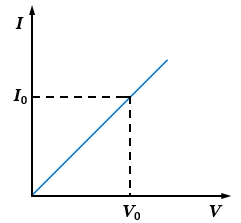# While studying the dependence of potential difference (V) across a resistor on the current (I) passing through it, in order to determine the resistance of the resistor, a student took 5 readings for different values of current and plotted a graph between V and I. He got a straight line graph passing through the origin. What does the straight line signify? Write the method of determining the resistance of the resistor using this graph.The straight-line graph of the potential difference $(V)$  across a resistor on the current $(I)$ passing through it signifies that the potential difference $(V)$ is directly proportional to the current $(I)$ flowing through it $(V\propto I)$.

The method to determine the resistance of the resistor using the graph is, read the current value in amperes at any point $(I_0)$, corresponding to a given voltmeter reading $(V_0)$.

From Ohm's law, we know that-

$V=I\times R$

For resistance it can be rearranged as-

$R=\frac {V}{I}$

Putting the value of $V=V_0$ and $I=I_0$, we get-

$R=\frac {V_0\ (value\ of\ potential\ difference\ at\ the\ point)}{I_0\ (value\ of\ current\ at\ that\ point)}$

Updated on: 10-Oct-2022

141 Views